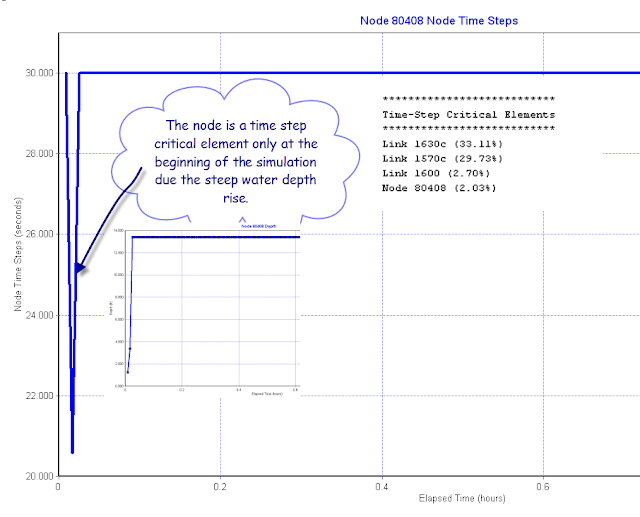## Node Time Step in SWWM 5

Subject:  Node Time Step in SWWM 5
The node time step in SWMM 5 is based on the maximum depth of the node, the last time step and the change in depth at the node between the current time step and the last time step.  It is calculated at the beginning of each time step in the dynamic wave solution of SWMM 5.  The maximum depth is the difference between the crown elevation of the node and the invert of the node. It is normally much less than the link  time step in SWMM 5 and is only important at the beginning of the simulation when the depth between the current node depth and the old node is large (Figure 1).  This is especially true if a hot start file is not used and the node starts out empty.   If the node time step is smaller than the link time steps it will be listed in the Table Time Step Critical elements (Figure 2).Figure 1.  Equations for the Node Time Step.Figure 2.  Node Time Step is critical at the beginning of the simulation.
Posted